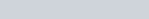ChemTalk

# What is Gibbs Free Energy?## Core Concepts

Gibbs free energy is an important value in thermodynamics that allows you to predict aspects of a chemical reaction. In this tutorial, we will learn why the Gibbs free energy equation is important and how to solve problems in which it is involved. We will also define what a spontaneous reaction is.

## Vocabulary

• Spontaneous reaction: a reaction that proceeds without any external force exerted on the system, such as temperature.
• Enthalpy (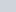): the overall heat in a reaction system.
• Entropy (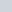): a value that describes the amount of disorder in a system, with a higher entropy value having more disorder. For example, a solid would be considered a system with order (low entropy), and a liquid or a gas would have more disorder (high entropy).
• Exergonic: when a reaction is spontaneous.
• Endergonic: when a reaction is nonspontaneous.

## Spontaneous Reaction Definition

A spontaneous reaction is a reaction that favors the formation of the products. Reactions that have a decrease in energy, and an increase in entropy, will be spontaneous. The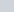value, defined below, will help predict if a reaction is spontaneous.

It is important to keep in mind, that a spontaneous reaction is one that occurs without any ongoing outside intervention or prolonged input of energy. Some spontaneous reactions, like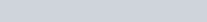will need a spark (a type of activation energy) to actually get the reaction started.

## What is Gibbs free energy?

Gibbs free energy () is a value that defines how spontaneous a reaction is, with a negative value meaning the reaction is spontaneous, and a positive value meaning the reaction is nonspontaneous. This value is the combination of enthalpy () and entropy ().

## What is the Gibbs free energy equation?

Gibbs free energy, enthalpy, and entropy can all be related in a simple equation. In this formula,,, andare expressed with a ∆(delta), indicating the change in these values over the reaction. The equation is as follows: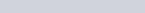In order for this equation to hold true, we must make two assumptions:

• The temperature remains constant throughout the reaction.
• The pressure remains constant throughout the reaction.

## How to solve a Gibbs free energy problem

Solving a Gibbs free energy problem can be easy if you are given each of the values stated in the formula. Let’s start with this reaction equation: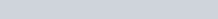Can we determine if this reaction is spontaneous? Here are the given conditions:

•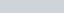•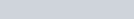•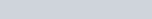Important: in order for this equation to hold true, the temperature must be converted to Kelvin. We can easily convert Celsius to Kelvin by adding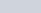to the temperature in celsius to get its value in Kelvin. In this case,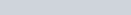. Now that we have converted the temperature, all we need to do is plug these values into the equation!Since the ∆G value is positive, this reaction is nonspontaneous, or an endergonic reaction.

## Further Examples

Now that we know how to to use the equation to find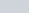, we can try more problems involving. Specifically, ifis given, we can solve for any of the other variables in the equation. Let’s try to solve for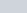in the following problem.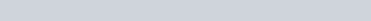Here are the given conditions:

••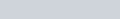•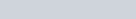We start by rearranging the equation to solve for.Now that we have solved for, we can plug our values into the equation. Similar to the last problem, we must convert temperature to Kelvin, which is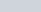again in this example.## Gibbs Free Energy Practice Problems

Problem 1

If a reaction has a negative change in enthalpy and is exergonic at certain temperatures, is the change in enthalpy positive or negative?

Problem 2

Consider the elemental synthesis of water: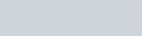•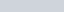•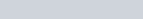•Calculate.

## Gibbs Free Energy Practice Problem Solutions

Problem 1

1: The change in enthalpy must be negative.

2: